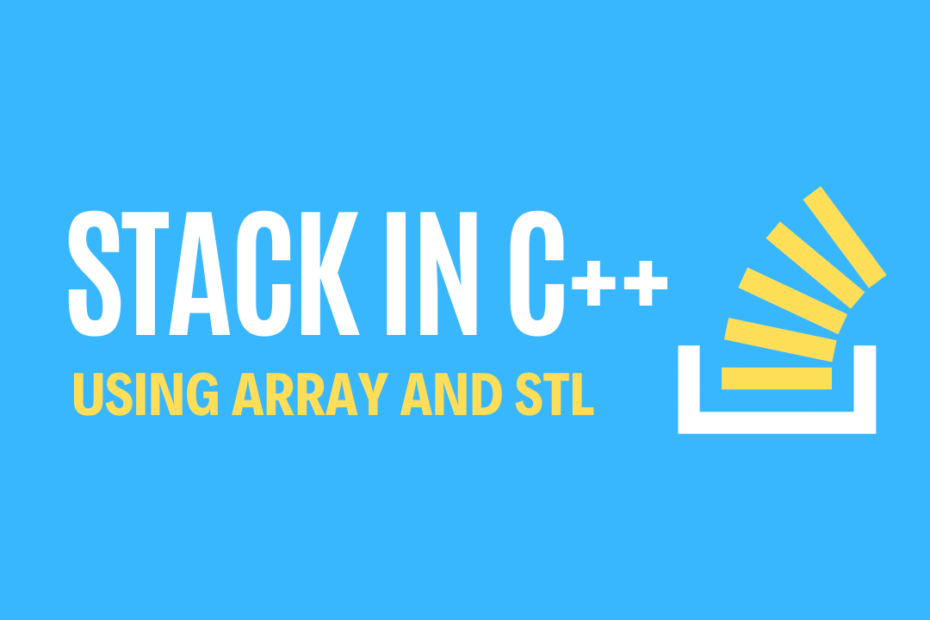# Stack Program in C++ using Array and STL

• DSA

I was brushing up my data structure skills and this post contains code for the Stack program using both Array and STL containers, the Stack follows the LIFO mechanism i.e Last In First Out, the element that was inserted last will also be the first to be deleted like a pile of chairs.

## Operations performed on a Stack

These are some of the main functions performed on a Stack

1. Push() – Insert data into the Stack
2. Pop() – Delete/remove data from the Stack
3. Top() – Displays the top most element of the Stack
4. isEmpty() – Stack is empty or not
5. isFull() – returns True if Stack is full
6. Display() – Prints all the contents of the Stack

## Stack Program using Array in C++

``````#include <iostream>
using namespace std;
//stack program
int stack, top = -1;
void push(int val)
{
if (top >= 100 - 1)
cout << Stack Overflow << endl;
else
{
top++;
stack[top] = val;
}
}
void pop()
{
if (top <= -1)
cout << Stack Underflow << endl;
else
{
cout << The popped element is  << stack[top] << endl;
top--;
}
}
void display()
{
if (top >= 0)
{
cout << Elements in Stack are:;
for (int i = top; i >= 0; i--)
cout << stack[i] <<" ";
cout << endl;
}
else
cout << Stack is empty;
}
int main()
{
int ch, val;
cout << 1) Push << endl;
cout << 2) Pop << endl;
cout << 3) Display << endl;
cout << 4) Exit << endl;
do
{
cout << Enter Your Choice:  << endl;
cin >> ch;
switch (ch)
{
case 1:
{
cout << Enter value to Insert:  << endl;
cin >> val;
push(val);
break;
}
case 2:
{
pop();
break;
}
case 3:
{
display();
break;
}
case 4:
{
cout << Exit << endl;
break;
}
default:
{
cout << Invalid Choice << endl;
}
}
} while (ch != 4);
return 0;
}``````

### Output

``````1) Push
2) Pop
3) Display
4) Exit
1
Enter value to Insert:
1
1
Enter value to Insert:
2
3
Elements in Stack are:2 1
4
Exit``````

## Stack Program using STL in C++

``````#include <iostream>
#include <stack>
using namespace std;

void display(stack<int> s)
{
while (!s.empty())
{
cout <<s.top()<<" ";
s.pop();
}
cout << '\n';
}

int main()
{
stack<int> s;
s.push(1);
s.push(2);
s.push(3);
s.push(4);
s.push(5);

cout <<"Contents in the Stack are: ";
display(s);
cout <<"Top of the Stack :  "<< s.top();
cout <<"\nSize of the Stack :  "<< s.size();
cout <<"\nPopped: ";
s.pop();
display(s);
return 0;
}
``````

### Output

``````Contents in the Stack are: 5 4 3 2 1
Top of the Stack : 5
Size of the Stack : 5
Popped: 4 3 2 1``````

This post is part of my #30DaysChallenge to write a blog post every day on what I learn. Cheers~ Abhiram Reddy